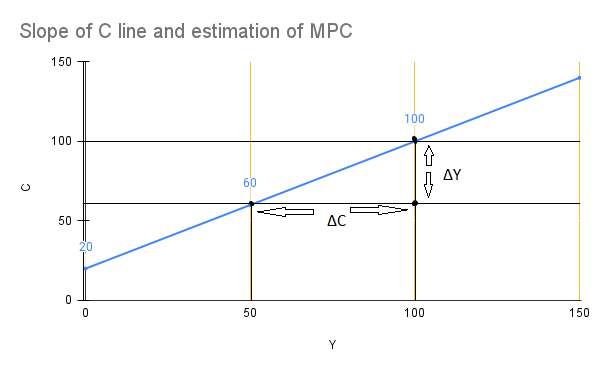# Consumption Function

A consumption function is a mathematical approach to finding out the relation between consumption and gross national income. The consumption expenditure is an essential component of aggregate demand. It is related to income(Y). Aggregate demand refers to the entire amount of money that individuals want to spend on goods and services produced in the economy at various levels of income.

If the income level (Y) is high then  C will also high and visa versa is also true. The income (Y) and consumption (C) is represented by a functional relation known as the consumption function. Therefore consumption function is a functional relationship between Y (income) and C (Consumption)

This relationship is helpful in understanding the behavior of consumption expenditure with respect to the income level. On observing the behavior of C and Y the experts of economics come up with the following essential points:

• There is some slightest level of C at the value of Y =0. This is known as autonomous consumption. This leads to the value of S in negative terms.
• Consumption is positively related to the value of income. The rise in the value of Y also increases the value of C and visa-versa is also true.
• Also, the whole increase in the value of Y is not converted in C. Some part of it is saved. It means the rate at which Consumption rises often lags behind the rate at which income increases. To completely understand the behavior of C with respect to Y consider the table mentioned below:
 Y(Income) C(Consumption) 0 20 50 60 100 100 150 140

This above-mentioned table  is the tabular presentation of the consumption function

i)The value of 20 is the minimum level of consumption. When income is zero, this demonstrates that even when Y=0, there is always some minimal amount of consumption. This minimum level of consumption during Y is zero is termed autonomous consumption.

ii)The value of C rises as the value of Y rises. It means that C is positively related to the value of  Y.

iii) When the value of Y rises from 0 to 50. At that time the value of C is also rising but its value is lower than Y. This shows saving. It means at a certain point the entire rise in Y is not turned into C. A part of it is saved.

Also read : Scope of Macroeconomics

## Diagrammatic Reprenstion

In the above graph, Consumption is represented on the Y-axis and income on the X-axis. There is a line Y which is inclined at an angle 45 . C line represents the consumption function. This line helps in observing the behavior of C with respect to Y.Line C starts from 20 which is the minimum level of income.The C line has a positive slope which is moving in the upward direction. More is the value of Y more will be the value of C. In the initial state, the value of Cis is higher than Y, Finally, its value starts lagging behind the value of Y.

## Relation With Marginal Propensity To Consume

The slope of line C is the representation of the C function. Let us elaborate it. Basically, it refers to the increase in the rate of C to the increase of Y . There is some proportion of additional income which goes into the consumption. This can be measured in terms of the rate of change in C to the rate of change in Y . This is termed Marginal prosperity to consume.

Marginal propensity to consume is the ratio of ΔC  to the ΔY .

### GraphFrom the above graph, the value of MPC is calculated as ΔC / ΔY =40/50=0.8

This shows out of every additional rupee of income the 80% part is spent for consumption.

The consumption function can be linear when MPC is constant.

## APC and MPC

The APC stands for average prosperity to consumption and MPC as marginal prosperity to consume. The average propensity to consume (APC)  is the ratio of total C(consumption) to the total Y ( income). The MPC, on the other hand, is the ratio of increased consumption( ΔC )to increased income (ΔY ).

### Mathematical representation of APC and MPC

APC=C/Y

MPC=ΔC/ΔY

as Y=150 and C=140 then

APC=140/150=0.933

as  ΔY=50 and ΔC =40

MPC=40/50=0.8

In essence, APC shows consumption per unit of total income and MPC shows the consumption per unit of additional income.

## Algebraic Representation of C function

The consumption function formula is expressed as  :

C=+bY

where C̅ is the minimum level of consumption. It is the value of consumption when Y is equal to 0. The b symbol is MPC.

The above algebraic equation helps us in calculating the different values of C corresponding to the different values of Y.

C=20+0.8Y(as given)

C when Y=0    =20+0.8(0)=20

C when Y=50  =20+0.8(50)=60

C when Y=100   =20+0.8(100)=100

C when Y=150   =20+0.8(150)=140

We come to the conclusion that the estimated values of consumption are exactly  same as mentioned in table we mentioned above in the article. Thus we can calculate the values of consumption once we know the values